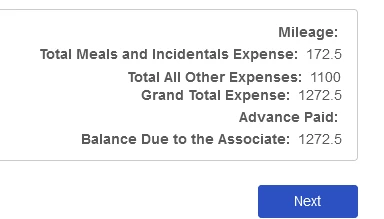Solved

# Formula Fixed Decimal

• 5 replies
• 7 views+6
• 45 replies

I have variables that sum up dollar amounts that I display in a label. I am trying to figure out how to add two-digit fixed decimal to whole dollar amounts, or amounts with only one decimal, but haven't been able to figure it out. Does anyone know how I could update the variable formulas to do this? I could use pad right, but I can't figure out how to evaluate the number to know whether to add ".00" or "0".

In my example below, I want it to show 172.50 for the second line, 1100.00 for the third.I know I could add read-only number fields for each value with 2 decimal places and create rules to display my variables instead, but that takes more than double the screen real estate and has extremely limited formatting options (no bold or italic, text size, etc..)

I would even be okay with editing the JSON and importing it in, but I don't know how to format a number that way either - everything I find shows how to do it with JS, but that went away after the Classic form.

icon

Best answer by AnneC 9 September 2022, 18:02

View original

### 5 replies

Userlevel 5+12

This video might be helpful: How to format currency in a label in Nintex Forms (Office365 or Nintex Workflow Cloud)+6

After spending (wasting?) an entire workday on it, I finally figured out how to do it without regex, just using the form functions. I created a text variable to format my control, [Form.TotalExpense] using this:

ifElse(substring(convertToString([Form].[TotalExpense]),
length(convertToString([Form].[TotalExpense]))-2,1)==".",
(convertToString([Form].[TotalExpense])+"0")
,
ifelse((not(contains(convertToString([Form].[TotalExpense]),"."))),
convertToString([Form].[TotalExpense])+".00",
convertToString([Form].[TotalExpense])))

Works beautifully!+6
Yes, I think I could have used a Replace function with a regular expression inside of my nested ifElse for my 'true' sections, but in this particular example, it seemed easier to just concatenate because there were only 2 possible search items and just one replace value for each. If I had more variable data, I think the opposite would be true.

Either way, thank you for getting me on the path to the answer, and for teaching me that a regex can be used in a replace.
Userlevel 5+12

Nice job! It works great.

I took it a step further using the video I previously shared and updated the formula to add a leading dollar sign (\$) and format with commas:

"\$" + replace(ifElse(substring(convertToString([Form].[TotalExpense]),length(convertToString([Form].[TotalExpense]))-2,1)==".",(convertToString([Form].[TotalExpense])+"0"),ifelse((not(contains(convertToString([Form].[TotalExpense]),"."))),convertToString([Form].[TotalExpense])+".00",convertToString([Form].[TotalExpense]))),"\B(?=(\d{3})+(?!\d))",",")+6
Wonderful! In my example, the requestor didn't want it 'cluttered up' with the dollar sign and commas, but I am sure at some point, someone will want it. Thank again for your help!